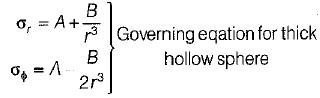Courses

# Test: Thin & Thick Walled Pressure Vessels - 1

## 10 Questions MCQ Test Topicwise Question Bank for Mechanical Engineering | Test: Thin & Thick Walled Pressure Vessels - 1

Description
This mock test of Test: Thin & Thick Walled Pressure Vessels - 1 for Mechanical Engineering helps you for every Mechanical Engineering entrance exam. This contains 10 Multiple Choice Questions for Mechanical Engineering Test: Thin & Thick Walled Pressure Vessels - 1 (mcq) to study with solutions a complete question bank. The solved questions answers in this Test: Thin & Thick Walled Pressure Vessels - 1 quiz give you a good mix of easy questions and tough questions. Mechanical Engineering students definitely take this Test: Thin & Thick Walled Pressure Vessels - 1 exercise for a better result in the exam. You can find other Test: Thin & Thick Walled Pressure Vessels - 1 extra questions, long questions & short questions for Mechanical Engineering on EduRev as well by searching above.
QUESTION: 1

### The area for circumferential stress calculation for the thin cylinder subjected to internal pressure is taken as

Solution:

Circumferential stress x resisting area = Pressure x projected area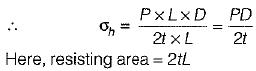QUESTION: 2

### A shell may be termed as thin if the ratio of thickness of the wall to the diameter of the shell is less than one to

Solution:

Explanation : T < Di/10 - Di/15 = Thin shell.

T > Di/10 - Di/15 = Thick shell.

i.e. 1/20

QUESTION: 3

### A cast-iron pipe of 750 mm diameter is used to carry water under a head of 60 m. Water is the thickness of pipe if the permissible stress is to be 20 MPa?

Solution:

Pressure = ρgH = 1000 x 9,81 x 60
= 588.6 kPa
∴ P = 0.5886 MPa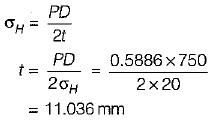QUESTION: 4

The initial hoop stress in a thin cylinder when it is wound with a wire under tension is

Solution:

A tube can be strengthened against the internal pressure by winding it with wire under tension and putting the tube wall in compression. As the pressure is applied, the resultant hoop stress produced is much less as it would have been in the absence of wire.

QUESTION: 5

The analysis of thick cylinders is usually based on Lame’s theory and for which assumptions are made as under:
1. The material of the cylinder is homogeneous and isotropic.
2. The material is stressed beyond elastic limits.
3. Yong’s modulus is the same in tension and compression.
4. Plane sections normal to the longitudinal axis of the cylinder remain plane after the application of pressure.

Which of the above assumptions are valid?

Solution:

The material is stressed within elastic limits.

QUESTION: 6

Hoop strain in case of thick cylinder subjected to internal pressure is given by

Solution: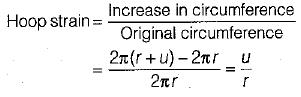QUESTION: 7

The error in calculation of hoop stress for a thin cylinder subjected to internal pressure if the ratio of internal diameter and thickness is 20

Solution:

Given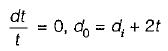Hoop stress at inner surface of thick cylinder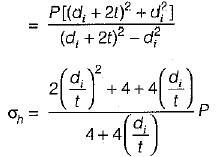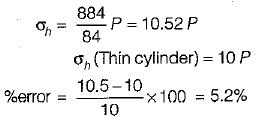QUESTION: 8

Variation of difference of radial and circumferential stress for a thick cylinder subjected to internal pressure follows:

Solution: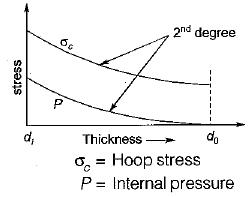QUESTION: 9

What is the increase of volume per unit volume of a thin-walled steel circular cylinder closed at both ends and subjected to a uniform internal pressure of 0.5 MPa? The wall thickness is 1.5 mm, the. radius 300 mm, and Poissson’s ratio = 0.33. Consider E - 200 GPa.

Solution: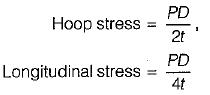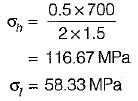Longitudinal strain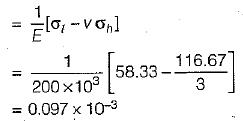Hoop strain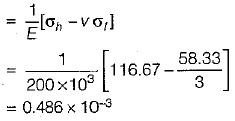Volumetric strain

= (0 .486 x 2 + 0.097) x 10-3
= 1.069x10-3

QUESTION: 10

In thick spherical pressure vessels, the variation of the stresses is

Solution: